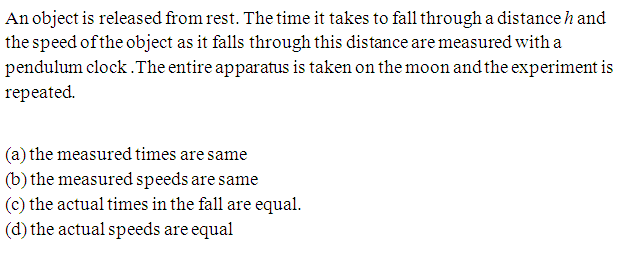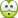# Simple pendulum

## Homework Statement## The Attempt at a Solution

Actual time taken by the object to fall by a distance h on earth = ##t = \sqrt{\frac{2h}{g}}##

Measured time by pendulum clock on earth = t

Actual time taken by the object to fall by a distance h on moon = ##t '= (\sqrt{6})\sqrt{\frac{2h}{g}}##

If actual time is t then time measured by pendulum clock on moon = ##\frac{t}{\sqrt{6}}##

Taking the above two factors into account , Time taken by the object to fall by a distance h on moon as measured by pendulum clock = t . Thus option a) is correct .

But the answer given is both a) and b) .

Now I wonder how can the measured speeds be same as speed ##v_{moon}## = (acceleration due to gravity on moon ) (time as measured by pendulum clock on moon ) .

Since ##g_{moon} = \frac{g}{6}## , speed after falling through 'h' as measured on moon ##v_{moon}## = ##\frac{v_{earth}}{6}## .

So, my doubt is , how is option b) correct and mistake in my reasoning .

I might be missing something .

Thanks

#### Attachments

Merlin3189
Homework Helper
Gold Member
.... Thus option a) is correct . ....
So, my doubt is , how is option b) correct and mistake in my reasoning .
If you say a) is correct, then how can b) not be correct? If the times are the same and the distances are the same, then the speed must be the same.

Your mistake is in calculating speed on moon.: g'=g/6 and t'=t√6
(Edit: just corrected above line)

Thanks for replying . I am feeling bit silly with this problem .

If the times are the same and the distances are the same, then the speed must be the same.

Hmm . But accelerations are different.

What is wrong with

##v_{moon}## = (acceleration due to gravity on moon ) (time as measured by pendulum clock on moon )
??
Your mistake is in calculating speed on moon.: g'=g/6 and t'=t√6

Sorry . I didn't get your point .

Merlin3189
Homework Helper
Gold Member
I don't feel so happy myself! My quick scribbles seemed to give the right answer, but when i worked through more carefully, I got a bit confused with real times and speeds and measured times and speeds!
anyhow -
a) and b) are both talking about measured speeds and times. We agree that on the moon, the measured time is the same as the measured time on earth.
So if you calculate the average speed for the falling object, you have the same measured time and the same measured distance, so must calculate the same measured speed.
Since h is not specified, it can be any height you like. This says that at all heights the measured speeds, times and distances match. Therefore we would also calculate the same measured acceleration on the moon as on earth.

Now you are saying, but we know the real acceleration on the moon is less, so we can calculate that the real speed on the moon is less. But for that we need to know the real time for the falls on the moon.
Now the real time of fall on the moon is √6 x the real time of fall on earth for that distance.
So if the real fall time on earth is t and the real final speed on earth is v=gt, then
$$on\ the\ moon\ the\ real\ final\ speed\ v'\ is\ \ \frac{g}{6}t√6 = \frac{gt}{√6} = \frac{v}{√6}$$ So the real speed on the moon is √6 times less than the real speed on earth.

That is consistent with our measured speed, because we are using a slow clock. Although it is really going √6 slower, our clock is going √6 slower, so we measure the same time as if it were going at v and our clock were correct
distance = real speed x real time or measured speed x measured time $$=\ \frac{v}{√6}\times{t√6}\ \ \ \ or\ \ \ \ {v}\times {t}$$

I still do not understand what is the flaw in
Measured speed ##v_{moon}## = (acceleration due to gravity on moon ) (time as measured by pendulum clock on moon ) .

@TSny , could you please see this .

TSny
Homework Helper
Gold Member
Now I wonder how can the measured speeds be same as speed ##v_{moon}## = (acceleration due to gravity on moon ) (time as measured by pendulum clock on moon ) .

Shouldn't this read ##v_{moon}## = (acceleration due to gravity on moon as measured by the pendulum clock on the moon ) (time as measured by pendulum clock on moon ) ?

•Vibhor
Shouldn't this read ##v_{moon}## = (acceleration due to gravity on moon as measured by the pendulum clock on the moon ) (time as measured by pendulum clock on moon ) ?

And how to measure acceleration due to gravity on moon by clock ?

Is it acceleration due to gravity on moon as measured by the pendulum clock = ##\frac{2h}{t^2}## ??

TSny
Homework Helper
Gold Member
And how to measure acceleration due to gravity on moon by clock ?

Is it acceleration due to gravity on moon as measured by the pendulum clock = ##\frac{2h}{t^2}## ??
Yes.

•Vibhor
Ah ! You just nailed the problem.

Quite interesting that the clock is measuring acceleration due to gravity on moon same as 'g'.

I feel there is a better , simpler way to analyze this problem . How would you think about this problem ?

TSny
Homework Helper
Gold Member
Here's a long-winded way:

Let ##t## be time as measured in true seconds. Let ##\tilde t## be time as measured by the pendulum clock.

At any location, a time interval ##\Delta \tilde t##measured by the pendulum clock is proportional to the number of cycles of swing of the pendulum during the time interval.

The number of cycles is ##\frac {\Delta t}{T}## where ##\Delta t## is the time interval measured in true seconds and ##T## is the period of the pendulum measured in true seconds.

Thus, ##\Delta \tilde t = k \frac {\Delta t}{T} ## for some constant k.

The pendulum clock is designed so that it measures seconds accurately when the pendulum is located on the earth. Therefore, on the earth we have ##\Delta \tilde {t}_E = \Delta t_E## which requires ##k = T_E##. Here, the subscript ##E## denotes on the earth.

Then at any location, ##\Delta \tilde t = \Delta t \frac{T_E}{T} = \Delta t \sqrt{ \frac{g}{g_E}}##, where ##g## is the acceleration of gravity at the location as measured using true seconds and ##g_E## is the acceleration of gravity on the earth also measured using true seconds.

Thus, a speed ##\tilde v## measured by the pendulum clock is ## \tilde v = \large \frac{dx}{d \tilde t} = \frac{dx}{d t} \frac{d t}{d \tilde t} = v \sqrt{ \frac{g_E}{g}}##.

Likewise, the acceleration ##\tilde a## of an object as measured by the pendulum clock at any location is seen to be

##\tilde a = a \large \frac{g_E}{g}##.

Hence, for the acceleration of gravity at any location as measured by the pendulum clock, we get

##\tilde g = g \frac{g_E}{g} = g_E##.

Thus, free-fall acceleration of an object as measured by the pendulum clock at any location is equal to the free-fall acceleration of an object on the earth .

Last edited:
•Vibhor
Then at any location, ##\Delta \tilde t = \Delta t \frac{T_E}{T} = \Delta t \sqrt{ \frac{g}{g_E}}##, where ##g## is the acceleration of gravity at the location as measured using seconds and ##g_E## is the acceleration of gravity on the earth also measured using seconds..

Is ##g## the correct value at the location or value as measured by clock ?

TSny
Homework Helper
Gold Member
It's the correct value. Quantities as determined by the pendulum clock have a tilde. Without the tilde, they are determined using an accurate standard clock. Hope I don't have too many typos.

•Vibhor
But then , from ##
\Delta \tilde t = \Delta t \frac{T_E}{T} = \Delta t \sqrt{ \frac{g}{g_E}}
## , we get
##\Delta \tilde t ≠ \Delta t ## , however they should be equal .

TSny
Homework Helper
Gold Member
If two events occur on the moon, the time interval will be ##\Delta \tilde t## as measured by the pendulum clock on the moon and ##\Delta t## will be the time interval between the same events as measured by an accurate clock. They will not agree since the pendulum is running slow.

•Vibhor
Ok . But then going by this same argument , how is it that in the original problem ## \Delta \tilde t = \Delta t## ?

TSny
Homework Helper
Gold Member
Ok . But then going by this same argument , how is it that in the original problem ## \Delta \tilde t = \Delta t## ?
In the original problem, you are comparing the time ##\Delta \tilde t_E## for an object to fall a distance h on the earth using a pendulum clock on the earth with the time ## \Delta \tilde t_M## for an object to fall a distance h on the moon using a pendulum clock on the moon. You are asked to show ##\Delta \tilde t_E = \Delta \tilde t_M ##.

##\Delta \tilde t_M = \Delta t_M \large \sqrt{ \frac{g_M}{g_E}}## relates the time ##\Delta \tilde t_M ## for an object to fall a distance h on the moon using a pendulum clock on the moon with the time ##\Delta t_M## for the object to fall a distance h on the moon using an accurate clock. ##g_M## and ##g_E## are accelerations of gravity on the moon and the earth, respectively, as measured by accurate clocks.

Last edited:
•Vibhor
Sorry . I was really slow in understanding your point .

Thank you very much